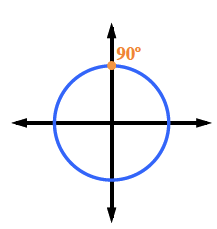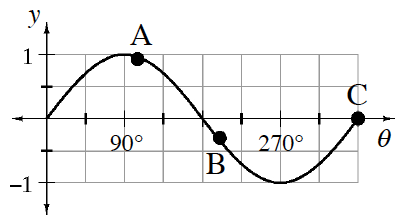### Home > INT3 > Chapter 9 > Lesson 9.1.3 > Problem9-31

9-31.

The graph at right represents the height above ground of a rider on The Screamer. Draw a unit circle and mark each of the corresponding locations of the labeled points. Then describe where the rider is located at each point. Homework Help ✎

Draw a unit circle. Notice that point A is slightly past $90^\circ$. Where is that on the unit circle?To locate point B, determine how the graph in the problem is scaled.